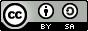# Machine Learning

John Samuel
CPE Lyon

Year: 2018-2019
Email: john(dot)samuel(at)cpe(dot)fr# Goals

1. Machine Learning
2. Deep Learning
3. Artificial Intelligence

# Formal definition

• Let X be the input feature space
• Let Y be the output feature space (of labels)
• The goal of classification algorithm (or classifier) is to find {(x1, y1),...,(xl, yk)}, i.e., assigning a known label to every input feature vector, where
• xiX
• yiY
• |X | = l
• |Y | = k
• l >= k

# 1. Machine Learning

## Classification

### Classifiers

• Classifying Algorithm
• Two types of classifiers:
• Binary classifiers assigning an object to any of two classes
• Multiclass classifiers assigning an object to one of several classes

# 1. Machine Learning

## Classification

### Linear Classifiers

• A linear function assigning a score to each possible category by combining the feature vector of an instance with a vector of weights, using a dot product.
• Formalization:
• Let X be the input feature space and xiX
• Let βk be vector of weights for category k
• score(xi, k) = xi.βk, score for assigning category k to instance xi. The category that gives the highest score is assigned as the category of the instance.

# 1. Machine Learning

## Classification

Let

• tp: number of true postives
• fp: number of false postives
• fn: number of false negatives

Then

• Precision p = tp / (tp + fp)
• Recall r = tp / (tp + fn)
• F1-score f1 = 2 * ((p * r) / (p + r))
• F1-score: best value at 1 (perfect precision and recall) and worst at 0.

# 1. Machine Learning

## Classification

### Multiclass classification

• Transformation to binary
• One-vs.-rest (One-vs.-all)
• One-vs.-one
• Extension from binary
• Neural networks
• k-nearest neighbours

# 1. Machine Learning

## Perceptron

• Algorithm for supervised learning of binary classifiers
• Binary classifier is a classifier which decides whether a given input belongs to a particular class or not
• Invented in 1958 by Frank Rosenblatt

# Perceptron: Formal definition

• Let y = f(z) be output of perceptron for an input vector z
• Let N be the number of training examples
• Let X be the input feature space
• Let {(x1, d1),...,(xN, dN)} be the N training examples, where
• xi is the feature vector of ith training example.
• di is the desired output value.
• xj,i be the ith feature of jth training example.
• xj,0 = 1

# Perceptron: Formal definition

• Weights are represented in the following manner:
• wi is the ith value of weight vector.
• wi(t) is the ith value of weight vector at a given time t.

# Perceptron: Steps

1. Initialize weights and threshold
2. For each example (xj, dj) in training set
• Calculate the weight: yj(t)=f[w(t).xj]
• Update the weights: wi(t + 1) = wi(t) + r. (dj-yj(t))xj,i
3. Repeat step 2 until the iteration error 1/s (Σ |dj - yj(t)|) is less than user-specified threshold.
4. s is the sample size and r is the learning rate.

# Feedforward neural network

• Connections between the nodes do not form a cycle
• Information moves from the input nodes, through the hidden nodes (if any) and to the output nodes.
• Information moves in only one direction, forward

# Backpropagation

• computes the gradient of the loss function with respect to the weights of the network for a single input-output example.
• works by computing the gradient of the loss function with respect to each weight by the chain rule

# Deep Learning

• uses multiple layers to progressively extract higher level features from the raw input.

# Convolutional Neural Networks

• Analysis of images
• Makes use of mathematical linear operation, convolution
• One input and one output layer
• Multiple hidden layers, consisting of convolutional layers